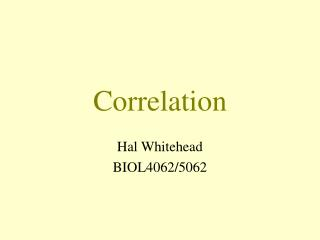# Correlation - PowerPoint PPT PresentationDownload PresentationCorrelation

Correlation
Download Presentation## Correlation

- - - - - - - - - - - - - - - - - - - - - - - - - - - E N D - - - - - - - - - - - - - - - - - - - - - - - - - - -
##### Presentation Transcript

2. The correlation coefficient • Tests • Non-parametric correlations • Partial correlation • Multiple correlation • Autocorrelation • Many correlation coefficients

3. The correlation coefficient

4. Linked observations: x1,x2,...,xny1,y2,...,yn  Mean:x = Σxi / ny = Σyi / n  Variance: S²(x)= Σ(xi-x)²/(n-1) S²(y)= Σ(yi-y)²/(n-1)  Standard Deviation: S(x) S(y) Covariance: S²(x,y) = Σ(xi-x) ∙ (yi-y) / (n-1)

5. Covariance: S²(x,y) = Σ(xi-x) ∙ (yi-y) / (n-1) Correlation coefficient (“Pearson” or “product-moment”): r = {Σ(xi-x) ∙ (yi-y) / (n-1) } / {S(x) ∙ S(y)} r = S²(x,y) / {S(x) ∙ S(y)}

6. The correlation coefficient: r = S²(x,y) / {S(x) ∙ S(y)} -1 ≤r≤ +1 If no linear relationship: r = 0 r2: proportion of variance accounted for by linear regression

7. r = -0.01

8. r = 0.38

9. r = -0.31

10. r = 0.95

11. r = 0.04

12. r = 0.64

13. r = -0.46

14. r = 0.99

15. r = -0.0

16. Tests on Correlation Coefficients

17. Tests on Correlation Coefficients • Assume: • Independence • Bivariate Normality

18. Tests on Correlation Coefficients • Assume: • Independence • Bivariate Normality

19. Tests on Correlation Coefficients • Assume: • Independence • Bivariate Normality • Then: z = Ln [(1+r)/(1-r)]/2 is normally distributed with variance 1/(n-3) And, if  (true population value of r) = 0 : r∙√(n-2) / √(1-r²) is distributed as Student's t with n-2 degrees of freedom

20. We can test: a) r≠ 0 b) r > 0 or r < 0 c) r = constant d) r(x,y) = r(z,w) Also confidence intervals for r

21. Are Whales Battering Rams?(Carrier et al. J. Exp. Biol. 2002) r= 0.75 (SE = 0.15) (95% C.I. 0.47-0.89) Tests: r≠ 0 : P = 0.0001 r > 0 : P = 0.00005 More sexually dimorphic species have relatively larger melons

22. Why do Large Animals have Large Brains?(Schoenemann Brain Behav. Evol. 2004) • Correlations among mammals • Log brain size with • Log muscle mass r=0.984 • Log fat massr=0.942 • Are these significantly different? t=5.50; df=36; P<0.01 Hotelling-William test • Brain mass is more closely related to muscle than fat

23. Non-Parametric Correlation

24. Non-Parametric Correlation • If one variable normally distributed • can test r=0 as before. • If neither normally distributed: • Spearman's rS rank correlation coefficient (replace values by ranks) or: • Kendall's τcorrelation coefficient • Use Spearman's when there is less certainty about the close rankings

25. Are Whales Battering Rams?(Carrier et al. J. Exp. Biol. 2002) r= 0.75 rS= 0.62 τ= 0.47

26. Partial Correlation

27. Partial Correlation • Correlation between X and Y controlling for Z r (X,Y|Z) = {r(X,Y) - r(X,Z)∙r(Y,Z)} √{(1 - r(X,Z)²)∙(1 - r(Y,Z)²)} • Correlation between X and Y controlling for W,Z r (X,Y|W,Z) = {r(X,Y|W) - r(X,Z|W)∙r(Y,Z|W)} √{(1 - r(X,Z|W)²)∙(1 - r(Y,Z|W)²)} n-2-c degrees of freedom (c is number of control variables)

28. Why do Large Animals have Large Brains?(Schoenemann Brain Behav. Evol. 2004) • Correlations among mammals • Log brain size with Log musclemass Controlling for Log bodymass r=0.466 Log fat mass Controlling for Log body mass r=-0.299 • Fatter species have relatively smaller brains and more muscular species relatively larger brains

29. Semi-partial Correlation Coefficient • Correlation between X & Y controlling Y for Z r (X,(Y|Z)) = {r(X,Y) - r(X,Z)∙r(Y,Z)} √(1 - r(Y,Z)²)

30. Are Whales Battering Rams?(Carrier et al. J. Exp. Biol. 2002) Correlation r= 0.75 Partial Correlation r (SSD,MA|L) = 0.73 Semi-partial Correlations r (SSD,(MA|L)) = 0.69 r ((SSD |L),MA) = 0.71

31. Multiple Correlation

32. Multiple Correlation Coefficient • Correlation between one dependent variable and its best estimate from a regression on several independent variables: r(Y∙X1,X2,X3,...) • Square of multiple correlation coefficient is: • proportion of variance accounted for by multiple regression

33. Autocorrelation

34. Autocorrelation • Purposes • Examine time series • Look at (serial) independence

35. Data (e.g. Feeding rate on consecutive days, plankton biomass at each station on a transect): 1.5 1.7 4.3 5.4 5.7 6.2 3.9 4.4 5.2 4.8 3.9 3.7 3.6 Autocorrelation of lag=1 is correlation between: 1.5 1.7 4.3 5.4 5.7 6.2 3.9 4.4 5.2 4.8 3.9 3.7 1.7 4.3 5.4 5.7 6.2 3.9 4.4 5.2 4.8 3.9 3.7 3.6 r = 0.508 Autocorrelation of lag=2 is correlation between: 1.5 1.7 4.3 5.4 5.7 6.2 3.9 4.4 5.2 4.8 3.9 4.3 5.4 5.7 6.2 3.9 4.4 5.2 4.8 3.9 3.7 3.6 r = -0.053 …….

36. Autocorrelation Plot (Correlogram)

37. Many Correlation Coefficients

38. Many Correlation Coefficients:[Behaviour of Sperm Whale Groups] Listwise deletion, n=40; P<0.10; P<0.05; uncorrected NGR25L SST SHITR LSPEED APROP SOCV SHR2 LFMECS LAERR NGR25L 1.00 SST 0.12 1.00 SHITR -0.21 -0.33* 1.00 LSPEED 0.10 -0.28+ 0.06 1.00 APROP -0.15 -0.34* 0.07 0.18 1.00 SOCV -0.05 0.08 -0.16 -0.01 -0.33* 1.00 SHR2 -0.18 -0.12 0.01 -0.20 0.19 -0.03 1.00 LFMECS 0.08 0.14 -0.13 -0.12 -0.22 0.29+ -0.18 1.00 LAERR -0.10 0.03 -0.21 -0.24 -0.02 0.24 -0.08 0.23 1.00 Expected no. with P<0.10 = 3.6; with P<0.05 = 1.8

39. Many Correlation Coefficients:[Behaviour of Sperm Whale Groups] Listwise deletion, n=40; P<0.10; P<0.05; Bonferronicorrected NGR25L SST SHITR LSPEED APROP SOCV SHR2 LFMECS LAERR NGR25L 1.00 SST 0.12 1.00 SHITR -0.21 -0.33 1.00 LSPEED 0.10 -0.28 0.06 1.00 APROP -0.15 -0.34 0.07 0.18 1.00 SOCV -0.05 0.08 -0.16 -0.01 -0.33 1.00 SHR2 -0.18 -0.12 0.01 -0.20 0.19 -0.03 1.00 LFMECS 0.08 0.14 -0.13 -0.12 -0.22 0.29 -0.18 1.00 LAERR -0.10 0.03 -0.21 -0.24 -0.02 0.24 -0.08 0.23 1.00 P=1.0 for all coefficients